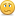Learning never exhausts the mind
Home >  Coding > C# ASP.Net MVC > C# Operators: Arithmetic, Comparison, Logical and more

# C# Operators: Arithmetic, Comparison, Logical and more

Published 11th August 2008 by

An operator is an element that is applied to one or more operands in an expression or statement. These are typically arithmetic or logical operators used for addition, subtraction, multiplication and division, or comparison.
Introduction to Programming with C# Series

We will also look at changing the behaviour of operators for custom classes and structs. This process is referred to as operator overloading.

## C# Operator List

The most common of the operators are the arithmetic and logic operators. These will be very familiar to you is you know other programming languages or mathematics.

## Arithmetic

OperatorActionExampleResult
+Additionz = 1 + 2z = 3
-Subtractionz = 1 - 2z = -1
*Multiplicationz = 2 * 2z = 4
/Divisionz = 22 / 7z = 3.142857
%Modulusz = 22 % 7z = 1
The modulus operator (%) computes the remainder after dividing its first operand by its second.

## Logic

OperatorActionExampleResult
&& Logical ANDtrue && false
true && true
false && false
false
true
false
||Logical ORtrue || false
true || true
false || false
true
true
false
!Logical NOTtrue && !falsetrue

## Increment and Decrement

OperatorActionExampleResult
++Incrementa=1;
a++;
a = 2
--Decrementa=1;
a--
a = 0;

## Relational

OperatorActionExampleResult
==Equalsx = 1;
x == 1
true
!=NOT Equalsx = 1;
x != 1
false
<Less thanx = 1;
x < 2;
true
>Greater thanx = 1;
x > 0;
true
<=Less than or equal tox = 1;
x <= 0
false
>=Greater than or equal tox = 1;
x >= 5
false

## Assignment

OperatorActionExampleResult
=Assignmentx = 1
a += 3;
a = 4;
-=Incremental Decrementa=1;
a -= 3;
a = -2;
*=Multiply bya=2;
a *= 4;
a = 8;
/=Divide bya=8;
a /= 2;
a = 4;
%=Modulus or Remaindera=8;
a %= 3;
a = 2;
&=Logical ANDx &= y" is equivalent to "x = x & y"
|=Logical OR"x |= y" is equivalent to "x = x | y"
<<=Left Shift"x <<= y" is equivalent to "x = x << y"
>>=Right Shift"x >>= y" is equivalent to "x = x >> y"

## Others

OperatorActionExampleResult
&Logical ANDif (false & ++i == 1)false
|Logical ORtrue | false
false | false
true
false
^Logical Exclusive XORfalse ^ false
false ^ true
true ^ true
false
true
false
~Bitwise Complementx = ~0x00000000x = 0xffffffff
<<Left Shift1 << 12
>>Right Shift-1000 >> 3-125
??Default Valueint y = x ?? -1;if x = null y = -1 else y = xConditional Operatorcondition ? expression if true : expression if false

In C#, operators can be overloaded as well as methods, a technique that allows custom data types to be manipulated in the same way as a normal data type.

Let's say you create a bank account class, for simplicity it will only contain a balance and holder name.

```public class bankAccount
{
decimal balance;
string holdersName;
}

public class Program
{
static void Main()
{
bankAccount testAccount1 = new bankAccount();
bankAccount testAccount2 = new bankAccount();

testAccount1.balance = 10.0;
testAccount1.holdersName = "Bob Smith";

testAccount2.balance = 20.0;
testAccount2.holdersName = "Jane Doe";
}
}```

If you wanted to add testAccount2 to testAccount1 you may be tempted to try:

`testAccount1 = testAccount1 + testAccount2`

or even

`testAccount1 += testAccount2`

You will find that the compiler will not let you add these together as it does not know how to handle the operators for this custom type. We can tell the C# compiler how to add two bank accounts together by overloading the operators.

```public class bankAccount
{
public decimal balance;
public string holdersName;

public static bankAccount operator +(bankAccount b1, bankAccount b2)
{
bankAccount temp = new bankAccount();
temp.balance = b1.balance + b2.balance;
temp.holdersName = b1.holdersName + " and " + b2.holdersName;
return temp;
}
}```

This will allow the use of the + operator on the bankAccount class. It will return a bankAccount, which contains the sum of the two balances and the two holders names concatenated.

All the other operators can be overloaded in the same way; all you need to do is provide your own logic within the method.

```testAccount1 = testAccount1 + testAccount2;

// testAccount1.ballance = 30
// testAccount1.holdersName = "Bob Smith and Jane Doe"```

Obviously, this is not how bank accounts are merged, but it gives an illustration of how operators can be overloaded for custom data types.

Tutorial Series

This post is part of the series Introduction to Programming with C#. Use the links below to advance to the next tutorial in the couse, or go back and see the previous in the tutorial series.

One thought on “C# Operators: Arithmetic, Comparison, Logical and more
•3rd February 2014 at 12:00 am

Thanks for the handy reference! I saved it to my bookmarks.
-Tim